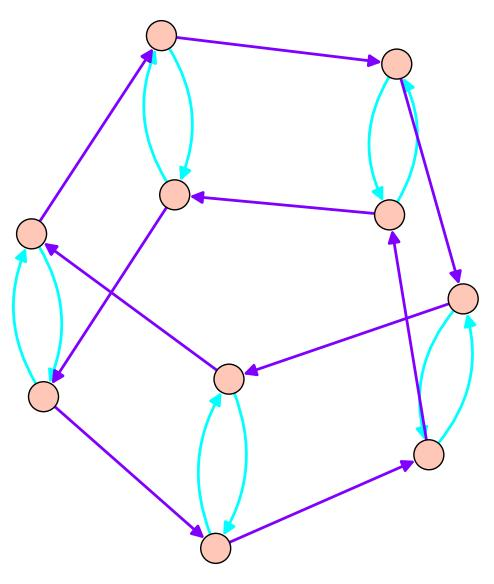Skip to main content
$$\newcommand{\id}{\mathrm{id}}$$ $$\newcommand{\Span}{\mathrm{span}}$$ $$\newcommand{\kernel}{\mathrm{null}\,}$$ $$\newcommand{\range}{\mathrm{range}\,}$$ $$\newcommand{\RealPart}{\mathrm{Re}}$$ $$\newcommand{\ImaginaryPart}{\mathrm{Im}}$$ $$\newcommand{\Argument}{\mathrm{Arg}}$$ $$\newcommand{\norm}{\| #1 \|}$$ $$\newcommand{\inner}{\langle #1, #2 \rangle}$$ $$\newcommand{\Span}{\mathrm{span}}$$

# 3.3: Cosets and Lagrage's Theorem

$$\newcommand{\vecs}{\overset { \rightharpoonup} {\mathbf{#1}} }$$

$$\newcommand{\vecd}{\overset{-\!-\!\rightharpoonup}{\vphantom{a}\smash {#1}}}$$

In this section, we'll prove Lagrange's Theorem, a very beautiful statement about the size of the subgroups of a finite group. But to do so,we'll need to learn about cosets.

Recall the Cayley graph for the dihedral group $$D_5$$ as generated by a flip and a rotation. Notice that the darker blue arrows look like two different 'copies' of $$\mathbb{Z}_5$$ sitting inside of the dihedral group. Likewise, the light arrow loops look like five copies of $$\mathbb{Z}_2$$. Both $$\mathbb{Z}_5$$ and $$\mathbb{Z}_2$$ are subgroups of $$D_5$$, generated by the rotation and flip respectively. These 'copies' of the subgroups that we see in the Cayley graph are examples of cosets.Dihedral group Cayley graph, generated by a flip and rotation. The Cayley graph for the dihedral group with generators given by a flip and a rotation.

Definition 3.3.0: Coset

Let $$H$$ be a subgroup of $$G$$, and $$H=\{h_1, h_2, h_3, \ldots \}$$. Then for any choice of $$g\in G$$, the coset $$gH$$ is the set $$\{gh_1, gh_2, \ldots \}$$.

These are precisely the 'copies' of the subgroups that we saw in $$D_5$$. The elements of $$D_5$$ can all be written as $$f^ir^j$$ with $$i\in \{0,1\}$$ and $$j\in \{0,1,\ldots,4\}$$. The rotation subgroup consists of the element $$R=\{e, r, r^2, r^3, r^4\}$$. Then $$R$$ has two distinct cosets, $$R$$ and $$fR=\{f, fr, fr^2, fr^3, fr^4\}$$. For any $$g$$ we choose, $$gR$$ is equal to one of these two cosets! Likewise, if we consider the subgroup $$F=\{e, f\}$$, there are five distinct cosets given by $$r^iF$$, where $$i\in \{0,1,2,3,4\}$$. Notice that the cosets evenly divide up the group; this isn't an accident!

Proposition 3.3.1

Suppose that $$H$$ is a subgroup of $$G$$. Let $$x, y\in G$$. Then either $$xH=yH$$ or $$xH$$ and $$yH$$ have no elements in common.

Proof 3.3.2

Suppose $$z\in xH$$ and $$z\in yH$$. In particular, there exist $$h_1, h_2\in H$$ such that $$z=xh_1=yh_2$$, and $$xh_1h_2^{-1}=y$$. We need to show that $$xH=yH$$, so take any $$h_3\in H$$ and consider $$yh_3\in yH$$. Then $$yh_3=xh_1h_2^{-1}h_3\in xH$$, since $$h_1h_2^{-1}h_3\in H$$. Thus, if $$xH$$ and $$yH$$ share any elements, then they are equal, and if they share no elements, they are tautologically disjoint!

We'll need one more piece of notation.

Definition 3.3.3: Order of a group

The order of a group $$G$$, written $$|G|$$, is the number of elements in $$G$$.

So the order of $$R\subset D_5$$ is $$|R|=5$$, and the order of the flip subgroup $$|F|=2$$. We can now prove Lagrange's Theorem!

Theorem 3.3.4: Lagrange's Theorem
Let $$H$$ be a subgroup of a finite group $$G$$. Then $$|H|$$ divides $$|G|$$.
Proof 3.3.5: Lagrange's Theorem

Notice that every element of the group $$G$$ shows up in some coset of $$H$$: since $$e\in H$$, we have $$g\in gH$$ for every $$g$$. Therefore, every element of the group shows up in exactly one coset of $$H$$. Also notice that every coset of $$H$$ has the same number of elements as $$H$$. (If the size of $$gH$$ were less than $$|H|$$, there would be have to be two different elements $$h_1, h_2\in H$$ with $$gh_1=gh_2$$. But cancelling the $$g$$'s gives $$h_1=h_2$$, a contradiction.)

Then the cosets of $$H$$ break up $$G$$ evenly into subsets of size $$|H|$$. Thus, $$|H|$$ divides $$|G|$$, as desired.

Exercise 3.3.6

Find all of the subgroups of the permutation group $$S_3$$ and the dihedral group $$D_5$$.

Exercise 3.3.7

Find a subgroup of the permutation group $$S_4$$ with twelve elements. What are it's cosets?

## Contributors

• Tom Denton (Fields Institute/York University in Toronto)